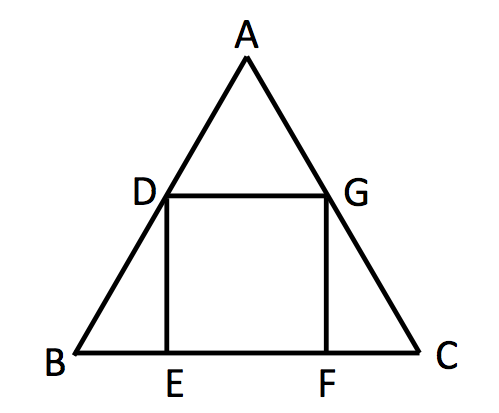# Square Inscribed Triangle

Geometry Level 4The area of Square $\Box DEFG$ is $a\sqrt{b}-c$, which is inscribed into an equilateral $\triangle ABC$.

With $a,b,c$ are all integers and $b$ square free. And The area of the equilateral triangle $\triangle ABC$ is $1$.

Evaluate $\dfrac{c-a}{b}$ to three decimal places.

×

Problem Loading...

Note Loading...

Set Loading...